# The Black Scholes Model and Implied Volatility

The Black Scholes model (BS model) is a mathematical model for pricing an option, either a call option or a put option. The standard BS model is only used to price European options, because it does not take into account that an option might be exercised before the maturity date. The BS model is very important to understand, because it is one of the key concepts used for option pricing.

## Key Assumptions of the Black Scholes Model

In the previous lecture, we focussed on the derivation process behind the BS model. The derived BS model assumes that the financial instruments have lognormal distributed prices. The BS model has been constructed by using this- and other key assumptions. Other key assumptions are:

• The underlying asset has no dividend payments.
• There is a constant risk-free rate.
• The asset is traded continuously over time.
• The asset is traded in a perfect market, in which there are no taxes and no transactions costs.
• Another very important assumption is that the BS model uses a constant volatility, which is a very important assumption and important to understand.

### No Dividend Payments

This assumption states that the underlying asset, such as a stock, does not pay dividends. This is important because dividends would reduce the stock’s price on the ex-dividend date, introducing an additional variable to model. By ignoring dividends, the model simplifies the asset’s price evolution.

### Constant Risk-Free Rate

The BS model assumes a constant risk-free rate, often represented by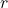, which is used to discount the option’s future payoffs. In reality, risk-free rates can vary over time, but assuming a constant rate simplifies calculations and allows for closed-form solutions.

The assumption that the asset is traded continuously over time allows the model to use stochastic calculus to derive the Black-Scholes equation. In the real world, trading is not continuous (e.g., markets close overnight), which can lead to gaps in pricing that the model doesn’t account for.

### Perfect Market

In a perfect market, there are no transaction costs or taxes, and buying/selling does not influence the asset’s price. This is clearly an idealization; in practice, transaction costs and taxes can significantly impact profitability and thus option pricing.

### Constant Volatility

This assumption posits that the asset’s volatility, often denoted by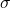, remains constant over the life of the option. Volatility is a measure of how much the asset price is expected to fluctuate. In reality, volatility can be time-varying and even “jumpy,” but assuming constant volatility allows for a closed-form solution to the Black-Scholes equation.

Each of these assumptions plays a role in simplifying the complexities of financial markets to a level where a closed-form solution like the Black-Scholes equation is possible. However, keep in mind that the simplifications also limit the model’s accuracy.

## Pricing of European Call- and Put Options

The pricing of European calls and puts is as follows. The pricing of a European call option on a non-dividend paying stock is given in Equation 1.

(1)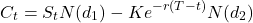The pricing of a European put option on a non-dividend paying stock is given in Equation 2.

(2)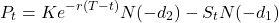In both Equation 1 and Equation 2:

•is the distribution function of the standardized normal distribution.
•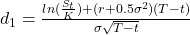•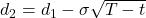These equations form the Black Scholes model, also known as the Black Scholes Merton model. Let’s get into examples for both a call option and a put option.

Example: Consider a European call that matures at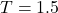. The exercise price is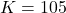, the current stock price is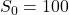, the volatility is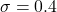and the risk-free rate is 5%.

Step 1: Compute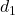and.

(3)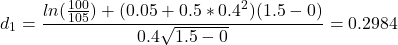(4)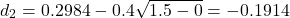Step 2: Determineand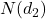. To do this, check a table for the z-statistic for the normal distribution.

(5)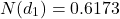(6)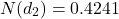Step 3: Compute the value of the call option at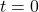.

(7)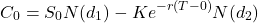(8)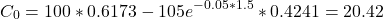Example: Consider a European put that matures at. The exercise price is, the current stock price is, , the volatility isand the risk-free rate is 5%.

Step 1: Computeand.

(9)(10)Step 2: Determineand. To do this, check a table for the z-statistic for the normal distribution.

(11)(12)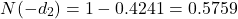Step 3: Compute the value of the put option at.

(13)(14)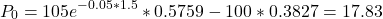As we can see in the formula, the volatility is an important input in the BS model. The higher the volatility is, the more expensive a call- or put option becomes. A higher volatility means that there is a larger probability that there will be a large price movement, which is a risk for the seller of the option contract. The BS model uses a fixed volatility. This volatility is called the implied volatility, which is the volatility that must be used in the BS model to obtain the option price that is observed in the market. In other words:

(15)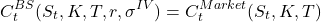## Implied Volatility Smile and Smirk

Empirical evidence shows us that, even though the BS model is very popular, the assumptions for a constant volatility does not hold. Empirical evidence shows us that the implied volatility depends on – for example – the time to maturity and on the exercise price. A short-dated option contract often results in a low implied volatility. On the other hand, a long-dated option contract often results in a high implied volatility. If we would plot the implied volatility against the exercise price, the BS model would suggest that the plot would be flat, since it should have a constant volatility. However, in reality we have a so-called volatility smile or volatility skew.

First, let’s elaborate on the volatility smile. A volatility smile is a graph shape in which the implied volatility is plotted against the strike price. Because of its shape it is called the volatility smile. Volatility smiles can be seen when various options with the same underlying asset and the same expiration date are compared for different strike prices. The smile pattern is commonly seen when equity options and options in the forex market are close to expiration. Other patterns occur in other circumstances, which will be elaborated on later on this section. In Figure 1, the implied volatility rises when the underlying asset of an option is further out of the money (OTM, a term to describe an option contract that will not directly give the buyer the right to buy the underlying asset below its current value or sell it above its current value) or in the money (ITM, a term used to describe an option contract that gives the buyer the right to buy the underlying asset below its current value or sell it above its current value), compared to at the money (ATM, when the option’s strike price is identical to the price of the underlying asset).

Demand drives the prices, which affect the implied volatility. ATM options are the most liquid contracts on the market. Therefore, their price is less volatile than the ones that are more ITM or OTM. The ITM- and OTM options are more driven by fear than greed. “People don’t pay much for flood insurance in the desert. But people pay considerable sums for flood insurance in coastal areas, specifically during hurricane season”. The psychology related to fear leads us more towards the skewed volatility graph in Figure 2, in which the graph shows more of a ‘smirk’ instead of a ‘smile’.

Near-expiration options might align more with the volatility smile, but long-term equity options and index options tend to align more with the volatility smirk. Just as with the volatility smile, the volatility smirk shows the difference in implied volatility between OTM-, ITM- and ATM options. The sentiment and the supply and demand schedules for the options with different strike prices affect the implied volatility. The smirk in Figure 2 is also called a reversed skew. The reverse skew pattern suggests that ITM calls and OTM puts are more expensive compared to OTM calls and ITM puts.

The reversed skew pattern is often explained in different ways. Here is a list of explanations:

• Leverage effect: One explanation for the volatility smirk can be attributed to the leverage effect, which is the inverse relationship between the price of an asset and its volatility. When an asset’s price declines, its volatility tends to increase. This is because as prices fall, the leverage of a company’s debt increases, making it riskier, and driving up the volatility. This effect causes the implied volatility of OTM put options to be higher than that of OTM call options, resulting in the volatility smirk.
• Market crashes and investor sentiment: Another contributing factor is the tendency for markets to experience larger declines during crashes than gains during rallies. Investors’ fear and risk aversion in times of market stress leads to a higher demand for downside protection through the purchase of OTM put options. This increased demand drives up the implied volatility for these options, again creating the volatility smirk.
• Market inefficiencies: Some researchers have argued that the volatility smirk may be a result of market inefficiencies, where traders do not price options correctly based on the historical distribution of asset returns. If traders systematically underestimate the likelihood of extreme negative events, the resulting market prices of options could exhibit a volatility smirk pattern.
• Jump risk and fat-tailed distribution: The distribution of stock returns is often assumed to follow a normal distribution in many options pricing models. However, empirical evidence suggests that stock returns exhibit fat tails and are prone to extreme events or jumps. The Black-Scholes model makes several simplifying assumptions, one of which is that stock returns follow a log-normal distribution. This means that the continuously compounded returns (the natural logarithm of the ratio of the ending price to the starting price) are normally distributed. Under this assumption, stock price movements are assumed to be continuous, with no sudden jumps, and are characterised by a symmetric, bell-shaped curve. When option prices are corrected to account for the increased probability of extreme negative events, the resulting implied volatilities exhibit a volatility smirk pattern.

In reality, however, empirical research has shown that the distribution of stock returns deviates from the normal distribution. Some key deviations include:
• Fat tails: The distribution of stock returns exhibits heavier tails compared to the normal distribution. This means that extreme events, both positive and negative, occur more frequently than what the normal distribution would predict. These fat tails can be attributed to events such as financial crises, natural disasters, or other occurrences that cause large price swings.
• Skewness: Stock returns are often found to be negatively skewed, meaning that the distribution is asymmetric with a longer tail on the negative side. This implies that large negative returns are more common than large positive returns. The normal distribution, on the other hand, is symmetric and does not account for this skewness.
• Kurtosis: The distribution of stock returns tends to have a higher kurtosis than the normal distribution, which means it has more probability mass in the tails and less in the center. In other words, extreme events are more likely, and moderate fluctuations are less likely compared to a normal distribution.
• Jump risk: The Black-Scholes model assumes that stock price movements are continuous and do not exhibit sudden jumps. However, real-world stock returns often involve discontinuous jumps due to unexpected news, political events, or other factors that cause abrupt changes in stock prices.
• Another reason is that ITM calls have become very popular, which drives up the price of the ITM call options. These call options often offer leveraged contracts, which increases the ROI (Return on Investment).

Another variant of the volatility smirk is the forward skew, shown in Figure 3. In the forward skew pattern, the implied volatility at lower strike prices are lower than the implied volatility at higher strikes. This suggests that OTM calls and ITM puts are in greater demand compared to ITM calls and OTM puts.